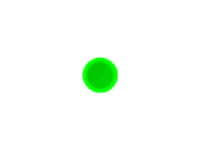### HexUsed by

This is the green "3D" ball used only in Alice and Bob and the Third Party.

FIXME: It appears to have more sound and animation, but is there a functional difference?

```<ball name="Hex"
shape="circle,24,0.25"
mass="8"
towermass="3"
strands="0"
walkspeed="0.05"
climbspeed="2.8"
speedvariance="0.2"
draggable="false"
detachable="false"
jump="0,0"
grumpy="false"

autoboundsunattached="false"

fallingattachment="false"

invulnerable="true"
>

<part name="body"
layer="1"
x="0" y="0"
image="IMAGE_BALL_HEX_BODY"
stretch="16,2,0.5"
scale="0.6"
/>

<!-- walking animations -->
<sinvariance freq="0.2" amp="0.1" shift="0.0">
<sinanim part="body"    state="walking" type="scale"     axis="x" freq="1.0" amp="0.1" shift="0"   />
<sinanim part="body"    state="walking" type="scale"     axis="y" freq="1.0" amp="0.1" shift="0.5" />
<sinanim part="body"    state="walking" type="translate" axis="y" freq="1.0" amp="2"   shift="0"   />
</sinvariance>

<!-- climbing animations -->
<sinvariance freq="0.8" amp="0.1" shift="0.0">
<sinanim part="body"    state="climbing" type="scale"     axis="x" freq="2.0" amp="0.1" shift="0"   />
<sinanim part="body"    state="climbing" type="scale"     axis="y" freq="2.0" amp="0.1" shift="0.5" />
<sinanim part="body"    state="climbing" type="translate" axis="y" freq="2.0" amp="2"   shift="0"   />
</sinvariance>

</ball>```